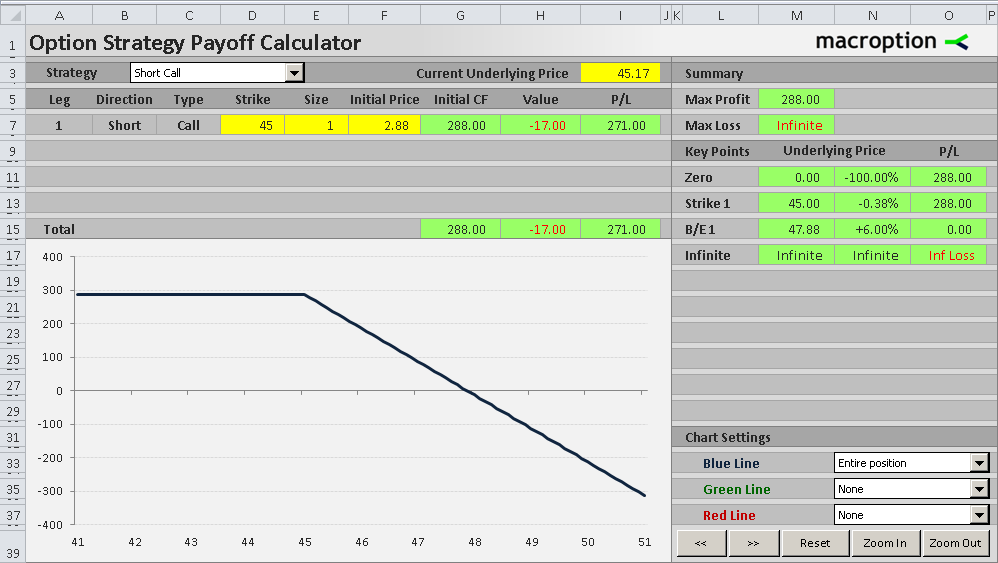# Short Call Payoff Diagram and Formula

A short call position is the opposite of a long call option position (the other side of the trade). You sell a call option and receive cash in the beginning. Then you either buy the option back or wait until expiration.

• you buy the option back for a lower price than what you sold it for, or if
• the option expires worthless or with intrinsic value lower than what you sold the option for.

## Short Call Payoff DiagramThe payoff diagram of a short call position is the inverse of long call diagram, as you are taking the other side of the trade. Basically, you multiply the profit or loss by -1.

For detailed explanation of the logic behind individual sections of the graph, see long call option payoff.

## Short Call Payoff Formulas

The formulas are the same as those for long call option strategy, only the profit or loss is multiplied by -1, because you are taking the other side of the trade.

There are again two components of the total profit or loss:

• The initial option price
• The value of the option at expiration

Only the signs are opposite compared to long call payoff.

Short call payoff per share = initial option price – option value at expiration

Option value at expiration = MAX ( 0 , underlying price – strike price )

Short call payoff per share = initial option price – MAX ( 0 , underlying price – strike price )

Short call payoff = ( initial option price – MAX ( 0 , underlying price – strike price ) ) x number of contracts x contract multiplier

## Short Call Break-Even Point

The formula for calculating short call break-even point is exactly the same as the one for long call break-even point:

Short call B/E = strike price + initial option price

For example, if you sell a 45 strike call option for 2.88 per share, the break-even price is 45 + 2.88 = 47.88 as in the example below. The trade is profitable if underlying price ends up below this point. If it gets above, the trade is losing money and the loss increases proportionally with underlying price.## Short Call Payoff Summary

• Short call strategy is directional and bearish – it generally makes money when the underlying price goes down (or doesn’t go up at least).
• It is also a short volatility strategy, as the value of a call option declines when volatility decreases, which means your short call position becomes more profitable.
• In other words, when selling a call option, you want the underlying security to not go up, or not move at all (assuming you’ve sold an out of the money call). You want the underlying price to end up below the strike price.
• Short call strategy has limited upside, equal to the cash you get when selling the call option in the beginning. This is the maximum you can gain from the trade.
• It has unlimited risk, because your total loss from the trade rises proportionally with the underlying price, which theoretically can go up infinitely.